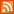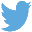Bucaro TecHelpHTTPS Encryption not required because no account numbers orpersonal information is ever requested or accepted by this siteCustom Search# Java Script Fahrenheit to Celsius Converter

Most of the world converted to the metric system of measurement decades ago, but here in the United States, only the scientific community uses metric measurements. The civilian population still uses the English system of measurement. Using two different systems of measurement is a problem. When the sign at the bank says the temperature is 24 degree's celsius, I don't know if that's warm or cool.

It's easy to do a conversion in your head if you are familiar with how the systems work. Water freezes at 32 degrees fahrenheit (F) or 0 degrees celsius (C). So the first thing we have to do to convert F to C is subtract 32. When converting C to F, you have to add 32.

Water boils at 212 degrees F or 100 degrees C, so the number of degrees between the freezing point and the boiling point of water is 180 degrees F or 100 degrees F. (To convert in your head just assume C degrees are twice as large as F degrees.)

So to convert F to C in your head, subtract 32 and divide the remainder by 2. To convert C to F in your head, double it and then add 32. This will give you the temperature within a few degrees of accuracy.

But who likes to do math in their head? In this article, I provide you with Java Script code for a Decimal to Fahrenheit to Celsius / Celsius to Fahrenheit Converter. I'll explain the code in detail so that you can have confidence modifying it for use on your website.

Fahrenheit to Celsius / Celsius to Fahrenheit Converter

The first thing you need is a form in which to enter the temperature you want to convert. Below is the html code for the form which you can paste into your webpage.

```Fahrenheit to Celsius / Celsius to Fahrenheit Converter<br>
<form name="tempform">
<input type="text" name="temp" style="text-align:right" size=4 maxlength=6>
<input type="button" value="To Celsius" onclick="ToC()">
<input type="button" value="To Fahrenheit" onclick="ToF()">
</form>
```

This form has no "Submit" button because the form will not be submitted to the server. All the processing will take place on the client side. Instead, two generic buttons, "To Celsius" and "To Fahrenheit" are provided. The onclick event of the "To Celsius" button calls a Java Script function named "ToC()". The onclick event of the "To Fahrenheit" button calls a Java Script function named "ToF()".

The form's text box has a style rule applied that causes the text to align with the right side of the text box so that it will look like a regular calculator. The "maxlength" attribute sets the largest number that can be entered into the text box to 6 digits.

Next you need the code for the Java Script "ToC()" and "ToF()" functions. Below is the Java Script code which you can paste into your webpage. You should paste the code into the <head> section of your webpage, but anywhere above the form code will work.

```<script language="JavaScript">

function ToC()
{
var strIn = document.tempform.temp.value;

if(isNaN(strIn))
{
}
else
{
var f = parseFloat(strIn);
var c = (f - 32) * 5/9;

var r = Math.round(c * 100)/100;
document.tempform.temp.value = r.toString();
}
}

function ToF()
{
var strIn = document.tempform.temp.value;

if(isNaN(strIn))
{
}
else
{
var c = parseFloat(strIn);
var f = (c * 9/5) + 32;

var r = Math.round(f * 100)/100;
document.tempform.temp.value = r.toString();
}
}
</script>
```

Note how the functions reference the form's text box. It uses "dot" notation to reference the document, then the form (by name), then the text box (by name), then the value in the text box.

 .menubtn { margin-top:10px; margin-left:50px; width:220px; opacity:1; border-style:solid; border-color:#009000; background-color:#66ff66; transition-property: opacity; transition-duration: 1s; transition-timing-function: ease-out; } .menubtn:hover { opacity:1; background-color:#e5ff23; } Menu - More Java Script ProgrammingRSS FeedFollow @Stephen Bucaro[Site User Agreement] [Privacy Policy] [Site map] [Search This Site] [Contact Form]
Copyright©2001-2021 Bucaro TecHelp 13771 N Fountain Hills Blvd Suite 114-248 Fountain Hills, AZ 85268